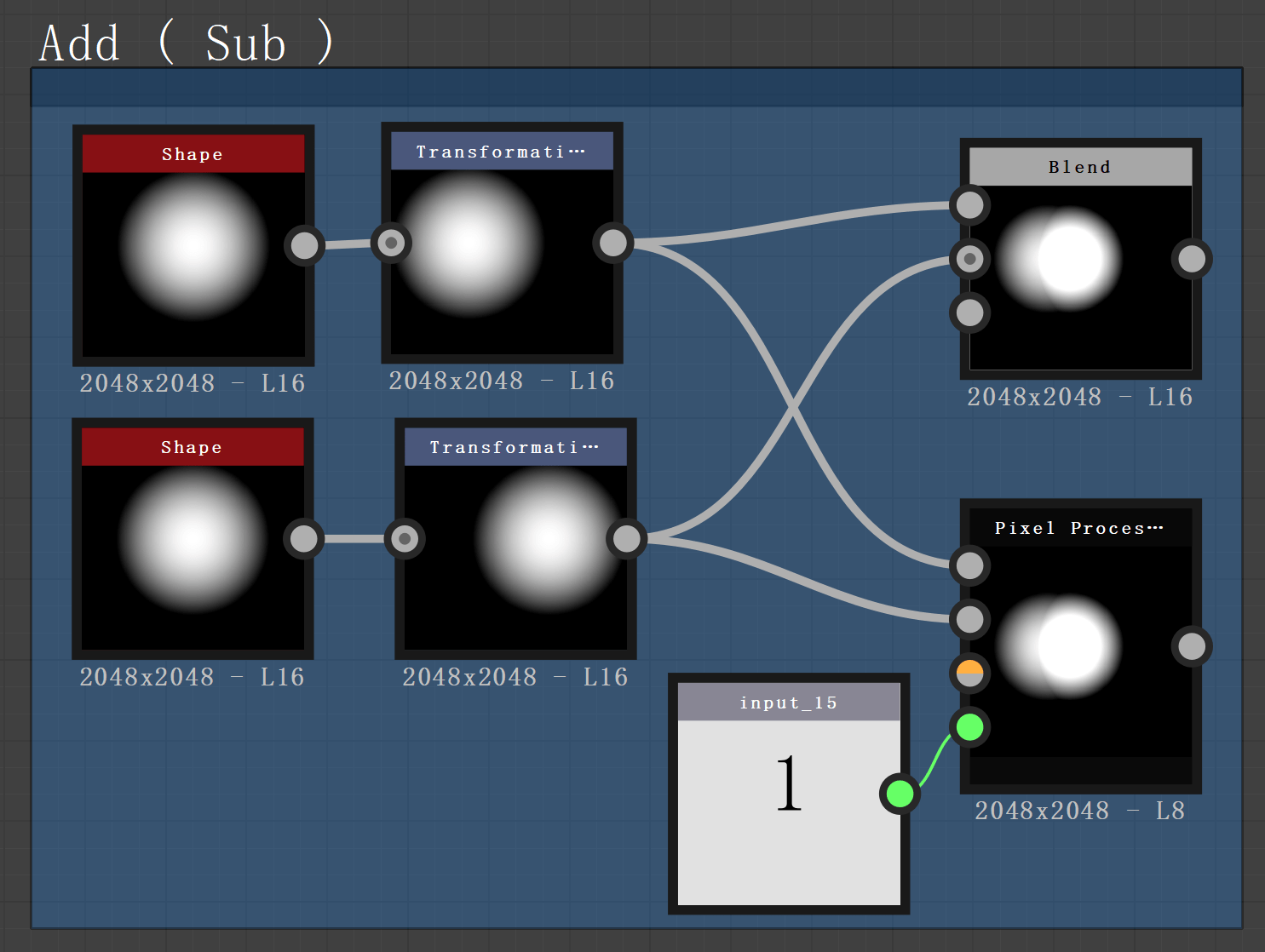# BLEND:ATOMIC NODE :SUBSTANCE DESIGNERBLEND:ATOMIC NODE :SUBSTANCE DESIGNER.

## 学习目标

1. 学习混合像素的 SD 所有 NODE 和功能的基本知识。
2. 理解Blend Node 的像素颜色合成式。
3. 把两个不同图片的 RGBA 用 Split 和 Merge 生成新的图片。
4. Channel Shuffle 和 Channel Mixer。

# Blend Mode 的种类。

• Copy
• Subtract
• Multiply
• Max(Lighten)
• Min(Darken)
• Switch
• Divide
• Overay
• Screen
• SoftLight

## Copy

Copy 是普通的 Linear interpolation 会混合两个信息。

V 值是 RGB 值 255 ， Float 值正确表示着 1.0。

RGB 加算混合(RGB 加算混合)是利用光的三原色来表现颜色的方式，所以这种结果是正确的。

Linear Interpolation law 以下线性插值法是可以填充两个数字间距的技术。

t = 0 就会回执 a。
t = 1，会返还 。``````public static Color LerpRGB (Color a, Color b, float t)
{
return ( a + (b - a) * t).rgba;
}``````

Pixel Processor 部分后面有说明用法，到时候再仔细看下。

``````float4 LinearDodge (float4 a, float4 b , float t)
{
return (a*t + b).rgba;
}``````

``````float4 Subtract (float4 a, float4 b , float t)
{
return lerp(a, (a-b), t);
}``````

## Multiply

``````float4 Multiply (float4 a, float4 b , float t)
{
return lerp(a, (a*b), t);
}``````

``````float4 AddSub (float4 a, float4 b , float t)
{
return  lerp(a, (b-1)+(b+a) , t);
}``````

## Max(Lighten)

``````float4 Lighten (float4 a, float4 b , float t)
{
return  max(a, b*t);
}``````

## Min(Darken)

``````float4 Darken (float4 a, float4 b , float t)
{
return min(a,  b * t);
}``````

## Divide

Color Mode

``````float4 Divide (float4 a, float4 b , float t)
{
return lerp(a ,(a/b), t);
}``````

Gray Mode

``````float Divide (float a, float b , float t)
{
float clampedA = clamp(a , 0.01 , 1)
float clampedB = clamp(b , 0.01 , 1)
return lerp(a ,(a/b), t);
}``````

## Difference

``````float4 Difference (float4 a, float4 b, float t)
{
return (abs(a - b*t));
}``````

## Overay

``````float4 Overlay (float4 a, float4 b)
{
float isLessOrEq = step(a, 0.5);
float4 cNew = lerp(2 * b*a, 1 - (1 - 2*(a - 0.5))*(1 - b), isLessOrEq);
cNew.a = 1.0;
return cNew;
}``````

## Screen

``````float4 Softlight(float4 a , float4 b , float t)
{
Float4 cNew =  (b < 0.5) ? (2.0 * a * b + a * a * (1.0 - 2.0 * b)) :
(sqrt(a) * (2.0 * b - 1.0) + 2.0 * a * (1.0 - b));
return (a , cNew , t);
}``````

## 其他一些算法…

ColorBurn

``````float4 ColorBurn (float4 a, float4 b, float t)
{
return (1 - (1 - a) / (b*t));
}``````

LinearBurn

``````float4 LinearBurn (float4 a, float4 b, float t)
{
return (a + b*t - 1);
}``````

HardLight

``````float4 HardLight (float4 a, float4 b, float t)
{
float4 valueB = b * t;
float isLessOrEq = step(valueB, .5);
float4 cNew = lerp(1 - (1 - a)*(1 - 2*valueB), 2*valueB*a, isLessOrEq);
return cNew;
}``````

VividLight

``````float4 VividLight (float4 a, float4 b, float t)
{
float4 valueB = b * t;
float isLessOrEq = step(valueB, .5);
float4 cNew = lerp(1 - (1 - a)/(2*(valueB - .5)), a/(1 - 2*valueB), isGreaterThen);
return cNew;
}``````

LinearLight

``````float4 LinearLight (float4 a, float4 b, float t)
{
float4 valueB = b * t;
float isLessOrEq = step(valueB, .5);
float4 cNew = lerp(a + 2*(valueB - .5), a + 2*valueB - 1., isLessOrEq);
return cNew;
}``````

PinLight

``````float4 PinLight (float4 a, float4 b, float t)
{
float4 valueB = b * t;
float isLessOrEq = step(b, .5);
float4 cNew = lerp(max(a, 2*(b - .5)), min(cBase, 2*b), isLessOrEq);
return cNew;
}``````

Exclusion

``````float4 Exclusion (float4 a, float4 b, float t)
{
return (.5 - 2 * (a - .5) * (b*t - .5));
}``````